Computer Organization and Structure

Homework #1

Due: 2004/10/12

1.      Given the following three functions:

I.                 A 2-bit-wide shifter takes two input signals,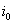and, and shifts them to two outputs,and, under the control of a shift signal. If this signal SHIFT is false, then the inputs are connected straight through to the outputs. If SHIFT is true, thenis routed toandshould be set to a.

II.              A 1-bit demultiplexer takes an input signal IN and shifts it to one of two outputs,and, under the control of a single SELECT signal. If SELECT is, then IN is connected through toandis connected to a. If SELECT is, then IN is connected through toandis connected to a.

III.            A 2-bit multiplexer takes two input signals,and, and shifts one of them to the single output OUT under the control of a 1-bit select signal. If the SELECT signal is false, thenis passed to OUT. If SELECT is true, thenis passed to OUT.

a.         Write truth tables.

b.        Write sum of products expressions.

c.         Draw logic schematics using AND, OR, and INVERT gates.

2.      Draw schematics for the following functions in terms of AND, OR, and inverter gates.

a.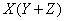b.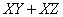c.d.e.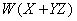3.      Draw the schematics for the following functions using NOR gates and inverters only:

a.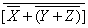b.4.      Prove the following simplification theorems using the first eight laws of Boolean algebra:

a.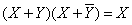b.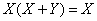c.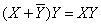d.5.      Simplify the following functions using the theorems of Boolean algebra. Write the particular law or theorem you are using in each step. For each simplified function you derive, how many literals does it have?

a.b.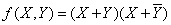c.d.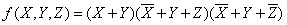e.6.      Consider a four-input Boolean function that is asserted whenever exactly two of its inputs are asserted.

a.         Construct its truth table.

b.        What is the function in sum of products form, using “little m” notation?

c.         What is the function in product of sums form, using “big M” notation?

d.        Use the Karnaugh map method to simplify the function in sum of products form.